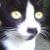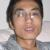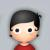## 17个新手常见Python运行时错误 热

1）忘记在 if , elif , else , for , while , class ,def 声明末尾添加 ：（导致 “SyntaxError ：invalid syntax”）

```if spam == 42
print('Hello!')```

2)使用 = 而不是 ==（导致“SyntaxError: invalid syntax”）

= 是赋值操作符而 == 是等于比较操作。该错误发生在如下代码中：

```if spam = 42:
print('Hello!')```

3）错误的使用缩进量。（导致“IndentationError：unexpected indent”、“IndentationError：unindent does not match any outer indetation level”以及“IndentationError：expected an indented block”）

```print('Hello!')
print('Howdy!')

if spam == 42:
print('Hello!')
print('Howdy!')

if spam == 42:
print('Hello!')```

4）for 循环语句中忘记调用 len() (导致“TypeError: 'list' object cannot be interpreted as an integer”)

```spam = ['cat', 'dog', 'mouse']
for i in range(spam):
print(spam[i])```

5）尝试修改string的值（导致“TypeError: 'str' object does not support item assignment”）

string是一种不可变的数据类型，该错误发生在如下代码中：

```spam = 'I have a pet cat.'
spam = 'r'
print(spam)```

```spam = 'I have a pet cat.'
spam = spam[:13] + 'r' + spam[14:]
print(spam)```

6）尝试连接非字符串值与字符串（导致 “TypeError: Can't convert 'int' object to str implicitly”）

```numEggs = 12
print('I have ' + numEggs + ' eggs.')```

```numEggs = 12
print('I have ' + str(numEggs) + ' eggs.')

numEggs = 12
print('I have %s eggs.' % (numEggs))```

7）在字符串首尾忘记加引号（导致“SyntaxError: EOL while scanning string literal”）

```print(Hello!')

print('Hello!)

myName = 'Al'
print('My name is ' + myName + . How are you?')```

8）变量或者函数名拼写错误（导致“NameError: name 'fooba' is not defined”）

```foobar = 'Al'
print('My name is ' + fooba)

spam = ruond(4.2)

spam = Round(4.2)```

9）方法名拼写错误（导致 “AttributeError: 'str' object has no attribute 'lowerr'”）

```spam = 'THIS IS IN LOWERCASE.'
spam = spam.lowerr()```

10）引用超过list最大索引（导致“IndexError: list index out of range”）

```spam = ['cat', 'dog', 'mouse']
print(spam)```

11）使用不存在的字典键值（导致“KeyError：‘spam’”）

```spam = {'cat': 'Zophie', 'dog': 'Basil', 'mouse': 'Whiskers'}
print('The name of my pet zebra is ' + spam['zebra'])```

12）尝试使用Python关键字作为变量名（导致“SyntaxError：invalid syntax”）

Python关键不能用作变量名，该错误发生在如下代码中：

`class = 'algebra'`

Python3的关键字有：and, as, assert, break, class, continue, def, del, elif, else, except, False, finally, for, from, global, if, import, in, is, lambda, None, nonlocal, not, or, pass, raise, return, True, try, while, with, yield

13）在一个定义新变量中使用增值操作符（导致“NameError: name 'foobar' is not defined”）

```spam = 0
spam += 42
eggs += 42```

14）在定义局部变量前在函数中使用局部变量（此时有与局部变量同名的全局变量存在）（导致“UnboundLocalError: local variable 'foobar' referenced before assignment”）

```someVar = 42
def myFunction():
print(someVar)
someVar = 100
myFunction()```

15）尝试使用 range()创建整数列表（导致“TypeError: 'range' object does not support item assignment”）

```spam = range(10)
spam = -1```

```spam = list(range(10))
spam = -1```

（注意：在 Python 2 中 spam = range(10) 是能行的，因为在 Python 2 中 range() 返回的是list值，但是在 Python 3 中就会产生以上错误）

16）不错在 ++ 或者 -- 自增自减操作符。（导致“SyntaxError: invalid syntax”）

```spam = 1
spam++```

```spam = 1
spam += 1```

17）忘记为方法的第一个参数添加self参数（导致“TypeError: myMethod() takes no arguments (1 given)”）

```class Foo():
def myMethod():
print('Hello!')
a = Foo()
a.myMethod()```

1#### 引用来自“李保银”的答案

```spam = ['cat', 'dog', 'mouse']
for i in range(spam):
print(spam[i])```

```spam = ['cat', 'dog', 'mouse']
for i in spam:
print i```

for i,e in enumerate(spam):
print i,e
0
p
0

```spam = ['cat', 'dog', 'mouse']
for i in range(spam):
print(spam[i])```

```spam = ['cat', 'dog', 'mouse']
for i in spam:
print i```

00Python 2.7.2

4

TypeError: range() integer end argument expected, got list.

11

KeyError: 'zebra'

13

NameError: name 'eggs' is not defined

0#### 引用来自“李保银”的答案

```spam = ['cat', 'dog', 'mouse']
for i in range(spam):
print(spam[i])```

```spam = ['cat', 'dog', 'mouse']
for i in spam:
print i```